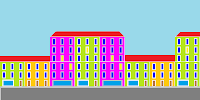## Friday, January 11, 2013

### Charlie

clg
penwidth 2
color (black ,-256^2/3)
circle 150,100,50
arc 150,100,13,13,0,3.14
circle 140,100,2
circle 170,100,2
arc 120,107,66,30,1.8,2.7
penwidth 1
arc 130,90,15,5,3.14*1.5,2
arc 163,90,15,5,6,1
arc 135,60,25,15,0,-5.14
arc 155,60,20,15,0,5.14

## Thursday, January 10, 2013

### Trigonometric Functions

graphsize 500,350
hip ={0,0,60,0,60,3,0,3,0,0}
fastgraphics
for n = 0 to pi*2 step .01
color black
circle 70,70,62
color white
circle 70,70,58
color black
stamp 70,70,1,-n,hip
rect 140,70,400,3
rect 140,250,400,3
color red
if cos(n)>0 then rect 70,70,60*cos(n),3
if cos(n)<0 then rect 70-60*cos(n+pi),70,60*cos(n+pi),3
color blue
if sin(n)>0 then stamp 70+cos(n)*60,70,sin(n),-pi/2,hip
if sin(n)<0 then stamp 70+cos(n)*60,70,sin(pi+n),pi/2,hip
color blue
circle n*50+140,50*sin(n+pi)+70,2
color red
circle n*50+140,50*cos(n+pi)+250,2
refresh
next n

### Normal Distribution

# Normal distribution simulation with mean=300 and standard deviation = 60

graphsize 600,600
sd=60
mean=300
dim bar(600)
for n = 0 to 99999
number = int(mean + sd*sqr(-2*log(rand))*cos(2*pi*rand))
bar[number]=bar[number]+1
plot number,500-bar[number]
next n

## Tuesday, January 8, 2013

### SpritesTownCars

clg
# For this program to work you need to copy the pictures and save in your disk/pen, and then change the path in imgload if necessary.

# This is the sprite sheet

spritedim 16
n=0
fastgraphics

# slicing vehicles from the sprite sheet
for y = 0 to 112.5 step 37.5
for x = 0 to 225 step 75
spriteslice n,x,y,75,37.5
n=n+1
next x
next y
pause 2

graphsize 600,300

#Backgroud image

# Placing vehicles
for n = 0 to 3
spriteplace n,int(rand*20)+n*100,260
spriteshow n
next n
for n = 4 to 7
spriteplace n,int(rand*20)+n*100-300,265
spriteshow n
next n
for n = 8 to 11
spriteplace n,int(rand*20)+n*100-700,275
spriteshow n
next n
for n = 12 to 15
spriteplace n,int(rand*20)+n*100-1100,280
spriteshow n
next n

# Makes sprites move
loop:
for n = 0 to 7
if n>-1 and n<4 then spritemove n,1.2,0
if n>3 and n<8 then spritemove n,.8,0
if spritex (n) = 600 then spritemove n,-600,0
next n
for n = 8 to 15
if n>7 and n<12 then spritemove n,-1.2,0
if n>11 and n<16 then spritemove n,-.8,0
if spritex (n) = 0 then spritemove n,600,0
next n
refresh
goto loop

### Charater count

# Gives a count of each character from t\$
t\$ = "Les Misérables, is a sung-through musical play based on the novel of the same name by French poet and playwright Victor Hugo. It has music by Claude-Michel Schönberg, original French lyrics by Alain Boublil and Jean-Marc Natel, with an English-language libretto by Herbert Kretzmer(...)"
font("arial",11,100)
t\$ = lower(t\$)
clg
long= length (t\$)
dim letters(255)

for n=1 to long
for x = 0 to 254
if chr(x)= mid(t\$,n,1)then letters[x]=letters[x]+1
next x
next n

#Frequency table
for x = 0 to 254
if letters[x]>0 then print chr(x)+" "+letters[x]
next x

# Bar chart (onlny for plain letters)
bar = 0
for x = 97 to 125
if letters[x]>0 then
Text 0,bar*12,chr(x)
rect 13,bar*12+6,letters[x],8
bar=bar+1
end if
next x

## Sunday, January 6, 2013

### Famous character 2

#With 3 lines we can make a famous character. Complete it and write your code as a comment.We will publish your attempts.
circle 150,200,90
circle 75,90,50
circle 225,90,50

## Friday, January 4, 2013

### Famous characters 1

#Complete the two famous characters. And post your code as a comment.

fastgraphics
color darkgreen
rect 0,0,300,300
color black
rect 55,120,50,6
Rem put the rest of the code here

refresh

color cor
for r = 0 to 1 step .001
next r
color black
next n
end subroutine

### Engine

graphsize 720,300
rod={2,1,2,-1,1,-2,-13,-2,-14,-1,-14,1,-13,2,1,2,2,1}
piston={-1,1,-1,-1,0,-2,5,-2,6,-3,13,-3,13,3,6,3,5,2,0,2,-1,1}
cylinder={-3,3,10,3,10,-3,-3,-3,-3,-4,11,-4,13,0,11,4,-3,4,-3,3}
cross={-1,4,1,4,1,1,4,1,4,-1,1,-1,1,-4,-1,-4,-1,-1,-4,-1,-4,1,-1,1,-1,4}
fastgraphics
for x = 0 to 10000 step .1
clg
color rgb(80,80,80)
stamp 480+35*cos(x),150,10,sin(x)/4,rod
color rgb(20,20,20)
circle 360,150,50
color rgb(120,120,120)
stamp 360,150,10,-x,cross
color rgb(80,80,80)
stamp 240+35*(-cos(x)),150,10,-sin(-x)/4+pi,rod
color rgb(20,20,20)
stamp 480+35*cos(x),150,10,piston
stamp 240+35*(-cos(x)),150,10,pi,piston
stamp 560,150,10,cylinder
stamp 160,150,10,pi,cylinder
color rgb(120*(sin(x))+125,127,120*(sin(x+pi))+125)
rect 61,121,35*(-cos(x))+49,59
rect 611-35*(-cos(x)),121,35*(-cos(x))+52,59
refresh
rem pause .01
next x

### Stampmaker

# Draw a shape then copy the array and use it for Stamps
graphsize 800,500
fastgraphics
print "Shape={";
oldx=0
oldy=0
n=2
dim shape(2)
init= true
fin = false
clickclear
decimal(0)

loop:
gosub drawgraph
circle mousex,mousey,3
color red
circle shape,shape,4
color black
refresh
clg
x=clickx/20-20
y=-clicky/20+12
if n>3 and shape[n-2]=shape and shape[n-1]=shapethen fin=true
# Checks for new click
if oldx<>clickx or oldy<>clicky then
if init = true then print x+","+y;
if init = false then
print ","+x+","+y;
n=n+2
end if
init= false
redim shape(n)
shape[n-2]= round (clickx,20)
shape[n-1]= round (clicky,20)
end if
oldx=clickx
oldy=clicky
if n > 3 then call draw (ref(shape),n,fin)
goto loop

subroutine draw(ref(shape),n,fin)
for p = 4 to n step 2
line shape[p-4],shape[p-3],shape[p-2],shape[p-1]
next p
if fin = true then
refresh
Print "}"
stamp 0,0,shape
refresh
print "Copy paste and run"
end
end if
end subroutine

drawgraph:
color black
for y= 0 to 480 step 20
for x= 0 to 800 step 20
circle x,y,2
next x
next y
line 0,240,800,240
line 400,0,400,480
return

function round(number,nearest)
if number%nearest<nearest/2 then
round = int (number/nearest)*nearest
else
round = int (number/nearest)*nearest+nearest
end if
end function

## Wednesday, January 2, 2013

### Mr Winky

# requires BASIC256 version 0.9.9.25 or later
# New functions (chord , arc , pie) showcase. Based on Jim's program

clg
color yellow
circle 150,150,150
color black
circle 100,100,20